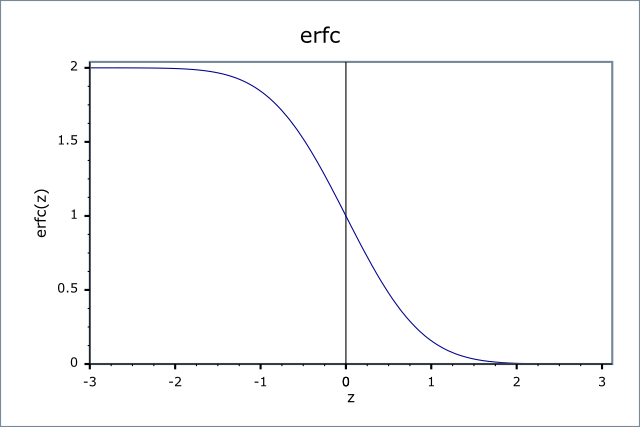#Boost C++ Libraries

...one of the most highly regarded and expertly designed C++ library projects in the world.

This is the documentation for an old version of boost. Click here for the latest Boost documentation.

#### Error Functions

##### Synopsis

```#include <boost/math/special_functions/erf.hpp>
```

```namespace boost{ namespace math{

template <class T>
calculated-result-type erf(T z);

template <class T, class Policy>
calculated-result-type erf(T z, const Policy&);

template <class T>
calculated-result-type erfc(T z);

template <class T, class Policy>
calculated-result-type erfc(T z, const Policy&);

}} // namespaces
```

The return type of these functions is computed using the result type calculation rules: the return type is `double` if T is an integer type, and T otherwise.

The final Policy argument is optional and can be used to control the behaviour of the function: how it handles errors, what level of precision to use etc. Refer to the policy documentation for more details.

##### Description
```template <class T>
calculated-result-type erf(T z);

template <class T, class Policy>
calculated-result-type erf(T z, const Policy&);
```

Returns the error function erf of z:```template <class T>
calculated-result-type erfc(T z);

template <class T, class Policy>
calculated-result-type erfc(T z, const Policy&);
```

Returns the complement of the error function of z:##### Accuracy

The following table shows the peak errors (in units of epsilon) found on various platforms with various floating point types, along with comparisons to the GSL-1.9, GNU C Lib, HP-UX C Library and Cephes libraries. Unless otherwise specified any floating point type that is narrower than the one shown will have effectively zero error.

Table 30. Errors In the Function erf(z)

Significand Size

Platform and Compiler

z < 0.5

0.5 < z < 8

z > 8

53

Win32, Visual C++ 8

Peak=0 Mean=0

GSL Peak=2.0 Mean=0.3

Cephes Peak=1.1 Mean=0.7

Peak=0.9 Mean=0.09

GSL Peak=2.3 Mean=0.3

Cephes Peak=1.3 Mean=0.2

Peak=0 Mean=0

GSL Peak=0 Mean=0

Cephes Peak=0 Mean=0

64

RedHat Linux IA32, gcc-3.3

Peak=0.7 Mean=0.07

GNU C Lib Peak=0.9 Mean=0.2

Peak=0.9 Mean=0.2

GNU C Lib Peak=0.9 Mean=0.07

Peak=0 Mean=0

GNU C Lib Peak=0 Mean=0

64

Redhat Linux IA64, gcc-3.4.4

Peak=0.7 Mean=0.07

GNU C Lib Peak=0 Mean=0

Peak=0.9 Mean=0.1

GNU C Lib Peak=0.5 Mean=0.03

Peak=0 Mean=0

GNU C Lib Peak=0 Mean=0

113

HPUX IA64, aCC A.06.06

Peak=0.8 Mean=0.1

HP-UX C Library Lib Peak=0.9 Mean=0.2

Peak=0.9 Mean=0.1

HP-UX C Library Lib Peak=0.5 Mean=0.02

Peak=0 Mean=0

HP-UX C Library Lib Peak=0 Mean=0

Table 31. Errors In the Function erfc(z)

Significand Size

Platform and Compiler

z < 0.5

0.5 < z < 8

z > 8

53

Win32, Visual C++ 8

Peak=0.7 Mean=0.06

GSL Peak=1.0 Mean=0.4

Cephes Peak=0.7 Mean=0.06

Peak=0.99 Mean=0.3

GSL Peak=2.6 Mean=0.6

Cephes Peak=3.6 Mean=0.7

Peak=1.0 Mean=0.2

GSL Peak=3.9 Mean=0.4

Cephes Peak=2.7 Mean=0.4

64

RedHat Linux IA32, gcc-3.3

Peak=0 Mean=0

GNU C Lib Peak=0 Mean=0

Peak=1.4 Mean=0.3

GNU C Lib Peak=1.3 Mean=0.3

Peak=1.6 Mean=0.4

GNU C Lib Peak=1.3 Mean=0.4

64

Redhat Linux IA64, gcc-3.4.4

Peak=0 Mean=0

GNU C Lib Peak=0 Mean=0

Peak=1.4 Mean=0.3

GNU C Lib Peak=0 Mean=0

Peak=1.5 Mean=0.4

GNU C Lib Peak=0 Mean=0

113

HPUX IA64, aCC A.06.06

Peak=0 Mean=0

HP-UX C Library Peak=0 Mean=0

Peak=1.5 Mean=0.3

HP-UX C Library Peak=0.9 Mean=0.08

Peak=1.6 Mean=0.4

HP-UX C Library Peak=0.9 Mean=0.1

##### Testing

The tests for these functions come in two parts: basic sanity checks use spot values calculated using Mathworld's online evaluator, while accuracy checks use high-precision test values calculated at 1000-bit precision with NTL::RR and this implementation. Note that the generic and type-specific versions of these functions use differing implementations internally, so this gives us reasonably independent test data. Using our test data to test other "known good" implementations also provides an additional sanity check.

##### Implementation

All versions of these functions first use the usual reflection formulas to make their arguments positive:

```erf(-z) = 1 - erf(z);

erfc(-z) = 2 - erfc(z);  // preferred when -z < -0.5

erfc(-z) = 1 + erf(z);   // preferred when -0.5 <= -z < 0
```

The generic versions of these functions are implemented in terms of the incomplete gamma function.

When the significand (mantissa) size is recognised (currently for 53, 64 and 113-bit reals, plus single-precision 24-bit handled via promotion to double) then a series of rational approximations devised by JM are used.

For ```z <= 0.5``` then a rational approximation to erf is used, based on the observation that erf is an odd function and therefore erf is calculated using:

```erf(z) = z * (C + R(z*z));
```

where the rational approximation R(z*z) is optimised for absolute error: as long as its absolute error is small enough compared to the constant C, then any round-off error incurred during the computation of R(z*z) will effectively disappear from the result. As a result the error for erf and erfc in this region is very low: the last bit is incorrect in only a very small number of cases.

For ```z > 0.5``` we observe that over a small interval [a, b) then:

```erfc(z) * exp(z*z) * z ~ c
```

for some constant c.

Therefore for ```z > 0.5``` we calculate erfc using:

```erfc(z) = exp(-z*z) * (C + R(z - B)) / z;
```

Again R(z - B) is optimised for absolute error, and the constant `C` is the average of ```erfc(z) * exp(z*z) * z``` taken at the endpoints of the range. Once again, as long as the absolute error in R(z - B) is small compared to `c` then ```c + R(z - B)``` will be correctly rounded, and the error in the result will depend only on the accuracy of the exp function. In practice, in all but a very small number of cases, the error is confined to the last bit of the result. The constant `B` is chosen so that the left hand end of the range of the rational approximation is 0.

For large `z` over a range [a, +∞] the above approximation is modified to:

```erfc(z) = exp(-z*z) * (C + R(1 / z)) / z;
```